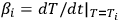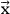# 1.1: Empirical Relationships and Specifics of Calculation Methods Used for Solving Non-Isothermal Kinetic Problems

$$\newcommand{\vecs}{\overset { \rightharpoonup} {\mathbf{#1}} }$$ $$\newcommand{\vecd}{\overset{-\!-\!\rightharpoonup}{\vphantom{a}\smash {#1}}}$$$$\newcommand{\id}{\mathrm{id}}$$ $$\newcommand{\Span}{\mathrm{span}}$$ $$\newcommand{\kernel}{\mathrm{null}\,}$$ $$\newcommand{\range}{\mathrm{range}\,}$$ $$\newcommand{\RealPart}{\mathrm{Re}}$$ $$\newcommand{\ImaginaryPart}{\mathrm{Im}}$$ $$\newcommand{\Argument}{\mathrm{Arg}}$$ $$\newcommand{\norm}{\| #1 \|}$$ $$\newcommand{\inner}{\langle #1, #2 \rangle}$$ $$\newcommand{\Span}{\mathrm{span}}$$ $$\newcommand{\id}{\mathrm{id}}$$ $$\newcommand{\Span}{\mathrm{span}}$$ $$\newcommand{\kernel}{\mathrm{null}\,}$$ $$\newcommand{\range}{\mathrm{range}\,}$$ $$\newcommand{\RealPart}{\mathrm{Re}}$$ $$\newcommand{\ImaginaryPart}{\mathrm{Im}}$$ $$\newcommand{\Argument}{\mathrm{Arg}}$$ $$\newcommand{\norm}{\| #1 \|}$$ $$\newcommand{\inner}{\langle #1, #2 \rangle}$$ $$\newcommand{\Span}{\mathrm{span}}$$

The solving of the kinetic problem consists of several steps. At the first step the inverse kinetic problem is solved by evaluating of Arrhenius parameters. However, the solution requires a series of experiments with different heating rates and determination of optimal experimental conditions:

$\ce{A_{s} -> B_{s} + C_{g}} \label{1.1}$

where As is the initial powdered solid reagent forming a flat layer, Bs is the solid reaction product located on the grains of the initial reagent, and Cg is the gaseous reaction product released into the environment. This process is referred to as quasi-one-stage, for reason that the reactions of the type of Equation \ref{1.1} have at least three stages and comprise the chemical reaction stage, heat and mass transfer. However, depending on the experimental conditions, one of the stages can be limiting. In our case, we believe the chemical reaction to be the rate-limiting stage. Let us assume that the dependence of this process on time and temperature is a single-mode thermoanalytical curve.

For such a process, the change in the reaction rate as a function of temperature can be described as follows:

$-\frac{d \alpha}{d t}=A e^{\frac{-E}{R T}} f(\alpha) \label{1.2}$

where A and E are the Arrhenius parameters, T is the temperature, and f(α) is some function of the conversion of the reaction characterizing its mechanism.

The conversion $$\alpha$$, according to Equation \ref{1.1}, is defined as the fraction of the initial reagent As that has reacted by time ti and changes from 0 to 1. It is worth noting that the conversion $$\alpha$$ can be calculated from both the TG and DSC data, as well as from differential thermogravimetry (DTG) data.

The so-called non-isothermal kinetic techniques are widely used owing to the apparent simplicity of processing experimental data according to the formal kinetic model described by Equation \ref{1.2}. Equation \ref{1.2} characterizes a single measurement curve.

As applied to experimental TA data, the kinetic model can be represented in the following form:

$-\frac{\mathrm{d} \alpha}{\mathrm{d} T_{T=T_{i}}}=\frac{A}{\beta_{i}} \exp \left(-\frac{E}{R T}\right) \alpha_{i}^{m}\left(1-\alpha_{i}\right)^{n} \label{1.3}$

where (1 - αi) is the experimentally measured degree of reaction incompleteness, Ti is the current temperature in kelvins (K),is the instantaneous heating rate (in our experiment, βi = β = constant), А is the preexponential factor, Е is the activation energy, m and n are the kinetic equation parameter .

Equation \ref{1.3} is easily linearized:

$\ln \left(-\frac{\mathrm{d} \alpha}{\mathrm{d} T}_{T=T_{i}}\right)=\ln \left(\frac{A}{\beta_{i}}\right)-\frac{E}{R T_{i}}+m \cdot \ln \alpha_{i}+n \cdot \ln \left(1-\alpha_{i}\right) \label{1.4}$

that is, it reduces to a linear least-squares problem.

The least-squares problem for Equation \ref{1.4} reduces to solving the following set of equations:

$C \vec{x}=\vec{b} \label{1.5}$

where C is the matrix of coefficients of Equation \ref{1.4} (Ci1 = 1/βi, Ci2 = -1/Ti, Ci3=lnαi, Ci4 = ln(1-α)), andis the vector of the sought parameters. Since the experimental data are random numbers, they are determined with a certain error.

Solving the problem in Equation \ref{1.5} involves certain difficulties.

The 1/T value changes insignificantly in the temperature range of reaction, therefore first and second column of matrix C are practically identical (up to a constant multiplier), and as a result matrix С is almost degenerate. Detailed description of this problem one can found in . To minimize uncertainty one should provide several experiments with different heating rates and calculate kinetic parameters using all experimental data.

The above arguments suggest only that the calculation of Arrhenius parameters using a single thermoanalytical curve is incorrect. The Netzsch Termokinetics Software uses a different approach, when the Arrhenius parameters are estimated using model-free calculation methods according to a series of experiments at different heating rates (see below).

The inverse kinetic problem thus solved does not guarantee the adequate description of experimental data. To verify the adequacy of the solution, it is necessary to solve the direct problem, that is, to integrate Equation \ref{1.3} and compare the calculated and experimental dependences in the entire range of heating rates. This procedure has been implemented in the NETZSCH Termokinetics, Peak Separation, and Thermal Simulation program packages with the help of NETZSCH. These programs and their applications are discussed in detail below.

Joint processing of experimental results obtained at different heating rates necessitates the constancy of the mechanism of a process, that is, the constancy of the type of f(α) function at different heating rates. Whether this condition is met can be verified by affine transformation of experimental curves, that is, by using reduced coordinates. To do this, one should select variables on the abscissa and ordinate so that each of them would change independently of the process under consideration. In addition, it is desirable that the relationship between the selected variables and experimental values be simple. These requirements are met by reduced coordinates. A reduced quantity is defined as the ratio of some variable experimental quantity to another experimental quantity of the same nature. As one of the variables, the conversion α is used, which is defined as the fraction of the initial amount of the reagent that has converted at a given moment of time. In heterogeneous kinetics, this variable is, as a rule, the conversion of the initial solid reagent.

If it is necessary to reflect the relationship between the conversion and time or temperature in the thermoanalytical experiment (at various heating rates), then the α is used as the ordinate and the reduced quantity equal to the ratio of the current time t or temperature T corresponding to this time to the time tα* it takes to achieve the desired conversion. For example, if the time or temperature required to achieve 50 or 90% conversion (α* = 0.5 or 0.9) is selected, the reduced quantities will be t/t0.5 (T/T0.5) or t/t0.9 (T/T0.9).

The above formalism pertains to the chemical stage of a heterogeneous process [4,5]. However, it should be taken into account that in general case heterogeneous process may involve heat and mass transfer, that is, the process may be never strictly one-step. The multistage character of a process significantly complicates the solution of the kinetic problem. In this case, a set of at least three differential equations with partial derivatives should be solved, which often makes the problem unsolvable. At the same time, experimental conditions can be found under which one of the stages, most frequently, the chemical stage, would be the rate-limiting stage of the process. Such experimental conditions are found in a special kinetic experiment.

1.1: Empirical Relationships and Specifics of Calculation Methods Used for Solving Non-Isothermal Kinetic Problems is shared under a CC BY-NC-SA 3.0 license and was authored, remixed, and/or curated by Igor V. Arhangel`skii, Alexander V. Dunaev, Irina V. Makarenko, Nikolay A. Tikhonov, & Andrey V. Tarasov via source content that was edited to conform to the style and standards of the LibreTexts platform; a detailed edit history is available upon request.